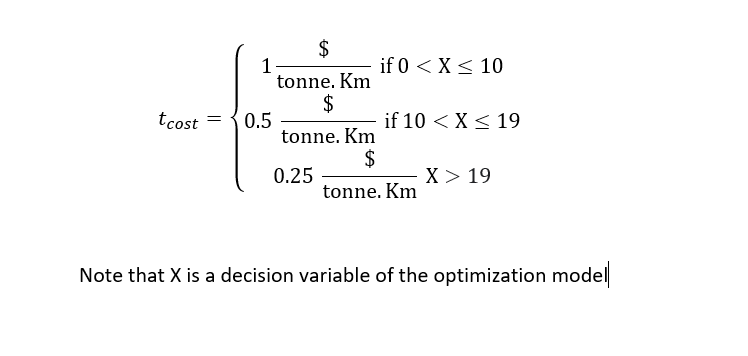•Gurobi Staff

Hi,

Without additional information on variables, constants, it is hard to determine what exactly you are trying to model. Maybe the stackexchange post on How to write if else statement in Linear Programming or the documentation on indicator constraints might be helpful.

Best regards,
Jaromił

•Hi,
thank you for responding

i'm trying to develop a network design model. i have two sets : facilities R  and products P

S_r_p : binary variable that is equal to 1 if facility r treat product p in P , 0 else

i'm trying to create a dependancy between two decision varaibles. i would like to maximize the number of products that each facilities treats.so i create another  binary decision variable called Y_r  that is equal to 1 if S_r_p of each facility for all the products is equal or superior than 3

i did not found the write program that describw what i want.this is my try

for k in R:
Z_r[k]= sum(S_r_p[k,j]for j in P)

for i in R:
m.addGenConstrIndicator(flag[i], True, Y_r[i] == 1 , name="indicator")

•Gurobi Staff

Hi,

Thank you for the clarification. This $$\texttt{if-then}$$-relationship is described in the stackexchange post How to write if else statement in Linear Programming and in your particular case would be given as

\begin{align} \sum_p S_p &\geq 3 - |P|\cdot(1-Y) \\ \sum_p S_p &\leq 2 + |P|\cdot Y \end{align}

This way, if $$\sum_p S_p \geq 3$$ then $$Y$$ has to be equal $$1$$ such that the second inequality is feasible. If $$\sum_p S_p \leq 2$$ then $$Y$$ has to be equal $$0$$ to make the first inequality feasible.

Maybe the post How Indicator constraint can be triggered with multiple variables? might be of interest to you.

Best regards,
Jaromił

•Hi,

thank you for responding
i have another question. i'm calculting transport cost of all nodes of a network.
the transport cost parameter (\$/weight) is not constant. it  depends on the weight of the shipement itself from  node a to node b for every arc in the network
my question is that i'm i supposed to consider this parameter as a decision vriable  ( because it changes fro every arc based on the shipement ) or as a simple parameter ?

Best Regards,
Ahmed,

•Gurobi Staff

Hi Ahmed,

If all weights are constant, you could then introduce a transport cost parameter for each of these weights which then again would be a constant. If you want to introduce the cost parameter as a function of weight, you will have to introduce it as an optimization variable. Please note that this then may result in a possibly nonconvex quadratic problem because you might have to multiply the cost variable with a node/arc variable. In this case you might have to set the NonConvex parameter to 2.

Best regards,
Jaromił

•Hi,
this is my case•Gurobi Staff

Hi Ahmed,

You can use piecewise-linear functions to model your function, see the documentation on piecewise-linear functions in objectives.

An alternative would be to model your function with indicator constraints and a partitioning constraint. Please note that Gurobi does not support strict inequality constraint, so you will have to introduce a positive tolerance $$\epsilon>0$$, e.g., $$\epsilon = 10^{-5}$$

First, you need to introduce 3 binary variables $$b_i \in \{0,1\}$$, 1 for each case. Then we say that

\begin{align} b_0 = 1 &\rightarrow 0+\epsilon \leq X \leq 10 \\ b_1 = 1 &\rightarrow 10+\epsilon \leq X \leq 19 \\ b_2 = 1 &\rightarrow 19+\epsilon \leq X \end{align}

The above means that if the binary variable $$b_0$$ equals $$1$$, then $$X$$ has to be in $$[\epsilon,10]$$.

Next, you have to make sure that exactly one of the cases is true. This can be done with the partitioning constraint

$b_0 + b_1 + b_2 = 1$

$$t_{cost}$$ can then be written as

$t_{cost} = b_0 \cdot 1 + b_1 \cdot 0.5 + b_2 \cdot 0.25$

Best regards,
Jaromił